Reach Us+44-1522-440391
Hypersurfaces of paraquaternionic space forms 1 | OMICS International
Journal of Generalized Lie Theory and Applications
All submissions of the EM system will be redirected to Online Manuscript Submission System. Authors are requested to submit articles directly to Online Manuscript Submission System of respective journal.

# Hypersurfaces of paraquaternionic space forms 1

Stere IANUS¸*1 and Gabriel Eduard VILCU2

1Faculty of Mathematics and Computer Science, University of Bucharest, Str. Academiei Nr.14, sector 1, 70109 Bucure¸sti, Romania E-mail: [email protected]

2Department of Mathematics and Computer Science, Petroleum-Gas University of Ploie¸sti, Bulevardul Bucure¸sti Nr. 39, 100680 Ploie¸sti, Romania E-mail: [email protected]

*Corresponding Author:
Stere IANUS
Faculty of Mathematics and Computer Science,
University of Bucharest, Str. Academiei Nr.14,
sector 1, 70109 Bucure¸sti, Romania
E-mail: [email protected]

Received date: December 10, 2007 Accepted Date: April 02, 2008

Visit for more related articles at Journal of Generalized Lie Theory and Applications

#### Abstract

In this paper we obtain some properties for real lightlike hypersurfaces of paraquaternionic space forms and give an example.

#### Introduction

It is well-known that the lightlike hypersurfaces are of interest in mathematical physics, owing to their extensive use in general relativity and electromagnetism. The general theory of lightlike submanifolds has been developed by Kupelli  and Bejancu-Duggal . In , the present authors and R. Mazzocco have begun the study of the real lightlike hypersurfaces of paraquaternionic manifolds. In this paper we obtain new properties for this kind of hypersurfaces and give some obstructions to the existence of lightlike hypersurfaces in paraquaternionic space forms.

#### Lightlike hypersurfaces

Let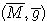be a (m+2)-dimensional semi-Riemannian manifold with index q ∈ {1, 2, . . . ,m+1} and let (M, g) be a hypersurface of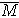, with g =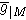. We say  thatM is a lightlike hypersurface of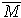if g is of constant rank m.

If we consider the vector bundle TM whose fibres are: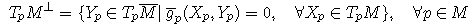then we can easily see that M is lightlike if and only if TM is a distribution of rank 1 on M.

Let S(TM) be the complementary distribution of TM in TM, which is called a screen distribution. This distribution is non-degenerate . Thus we have direct orthogonal sum decomposition TM = S(TM) ⊥ TM. On the other hand, if S(TM)? is the orthogonal complementary vector bundle to S(TM) in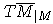,we have direct orthogonal sum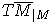= S(TM)S(TM)?.

From  we know that, if (M, g, S(TM)) is a lightlike hypersurface of M, then there exists a unique vector bundle ltr(TM) of rank 1 over M so that for any non-zero section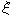of TM on a coordinate neighborhood U ⊂ M, there exists a unique section N of ltr(TM) on U satisfying: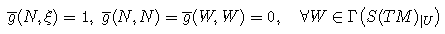The vector bundle ltr(TM) is called the null transversal vector bundle of M with respect to S(TM). Thus, we have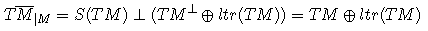Let (M, g, S(TM)) be a lightlike hypersurface of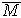. If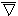is the Levi-Civita connection on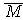, then we have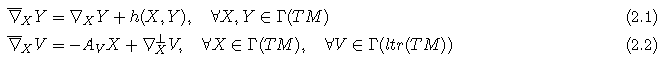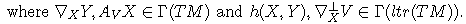∇ is a symmetric linear connection on M called an induced linear connection, ∇⊥ is a linear connection on the null transversal bundle ltr(TM), h is a Γ(ltr(TM))-valued symmetric bilinear form and AV is the shape operator of M with respect to V . If {ε,N} is a pair of sections on U ⊂ M as above, then we define a symmetric F(U)-bilinear form B and a 1-form τ on U by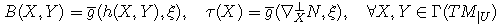Thus, from (2.1) and (2.2), locally we have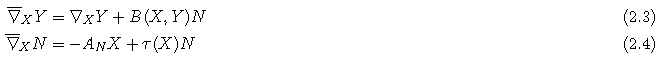If we denote by P the projection of TM on S(TM), we have the decompositions: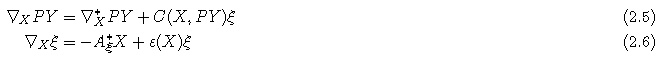where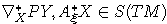and ε is a 1-form on U. Moreover, it is easy to see that ε = −τ .

#### Real lightlike hypersurfaces of paraquaternionic manifolds

Let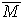be a smooth manifold. We say that a rank-3 subbundle σ of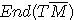is an almost paraquaternionic structure on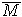if a local basis {J1, J2, J3 } exists on sections of σ, such that for all α ∈ {1, 2, 3} we have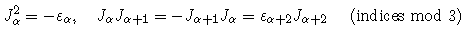where ε1 = 1, ε2 = ε3 = −1.

Let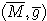be a semi-Riemannian manifold and σ an almost paraquaternionic structure on M. The metric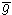is said to be adapted to the paraquaternionic structure σ if it satisfies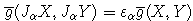for all α ∈ {1, 2, 3} and for all vector fields X, Y on M and any local basis {J1, J2, J3 } of σ, where ε1 = 1, ε2 = ε3 = −1. Moreover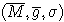is said to be an almost hermitian paraquaternionic manifold. Moreover, if the Levi-Civita connection of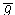satisfies the following conditions for all α ∈ {1, 2, 3}:for any vector field X on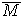, !1, !2, !3 being local 1-forms over the open for which {J1, J2, J3 } is a local basis of σ and ε1 = 1, ε2 = ε3 = −1, then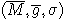is said to be a paraquaternionic K¨ahler manifold (see ).

Let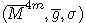be an almost hermitian paraquaternionic manifold and let (M, g) be a real lightlike hypersurface of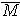. We remark that we can choose the screen distribution S(TM) such that it contains Jα(TM) as a vector subbundle, because we have: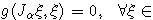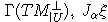are tangent to M and thus Jα(TM) is a 3-rank distribution on M such that Jα(TM) \ TM = {0}.

If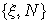is a pair of sections on U ⊂ M as in above section, then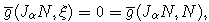and we deduce that JαN 2 Γ(S(TM)). It is easy to see that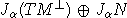is a vector subbundle of S(TM) of rank 6. Then there exists non-degenerate distribution D0 on M such that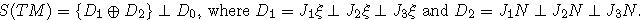We remark that the distribution D0 is invariant with respect to Jα for all α 2 {1, 2, 3} (see ). We consider now the local lightlike vector fields: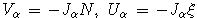for all α 2 {1, 2, 3}. Then any local vector field on M is eXpressed as follows: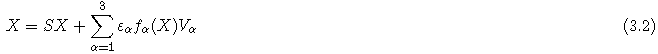where S is the projection on the almost paraquaternionic distribution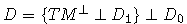and fα are 1-forms locally defined on M by: fα(X) = g(X,Uα), for all α 2 {1, 2, 3}.

By using (3.2) we derive: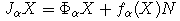where ΦαX are the tangential components of JαX for all α 2 {1, 2, 3}.

Theorem 3.1. Let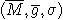be a paraquaternionic K¨ahler manifold and let M be a lightlike hypersurface of M. Then M is totally geodesic if and only if: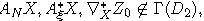for all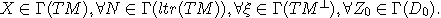Proof. By using (2.3), (2.4) and (3.1) we obtain for any X 2 Γ(TM) and Z0 ∈ Γ(D0):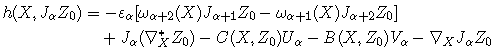The proof is now complete, since by the definition of a lightlike hypersurface, M is totally geodesic if and only if: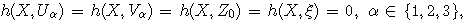for all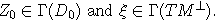Theorem 3.2. Let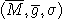be a paraquaternionic K¨ahler manifold and let M be a lightlike hypersurface of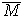. Then D0 is integrable if and only if: C(X, Y ) = C(Y,X), C(X, JαY ) = C(Y, JαX), B(X, JαY ) = B(Y, JαX) for all X, Y 2 Γ(D0) and α 2 {1, 2, 3}.

Proof. D0 is integrable if and only if: 8X, Y 2 Γ(D0) ) [X, Y ] 2 Γ(D0) and from the general decomposition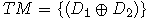? D0 ? TM} we deduce that D0 is integrable if and only if: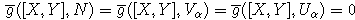for all X, Y 2 Γ(D0), N 2 Γ(ltr(TM)) and α 2 {1, 2, 3}. For X, Y 2 Γ(D0) and N 2 Γ(ltr(TM)), by using (2.5) we deduce: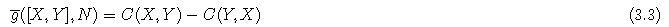From (2.5) and (3.1), we obtain: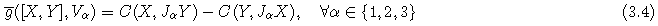and similarly we deduce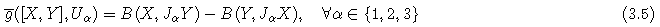The proof is now complete from (3.3), (3.4) and (3.5).

Corollary 3.1. Let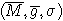be a paraquaternionic K¨ahler manifold and let M be a totally geodesic lightlike hypersurface of M. Then the distribution D is parallel.

#### Non-existence of real lightlike hypersurfaces in paraquaternionic space form

Let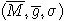be a paraquaternionic K¨ahler manifold and let X be a non-lightlike vector on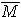. Then the 4-plane spanned by {X, J1X, J2X, J3 X}, denoted by PQ(X), is called a paraquaternionic 4-plane. Any 2-plane in PQ(X) is called a paraquaternionic plane. A paraquaternionic K¨ahler manifold is said to be a paraquaternionic space form if its paraquaternionic sectional curvatures are equal to a constant.

We recall now the following technical results .

Lemma 4.1. Let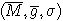be a paraquaternionic K¨ahler manifold and let M be a lightlike hypersurface of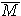. Then we have for all α ∈ {1, 2, 3}: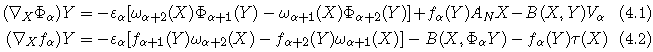(indices mod 3) for all X, Y ∈ Γ(TM).

Let (M, g) be a lightlike hypersurface of a semi-Riemannian manifold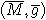. The null sectional curvature of M at p ∈ M with respect to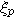, is the real number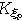(M) defined by: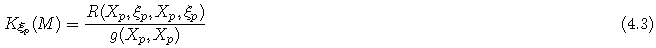where Xp is non-null vector in TpM.

Theorem 4.1. There are no real lightlike hypersurfaces with positively or negatively null sectional curvature of paraquaternionic space forms.

Proof. Let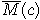be a paraquaternionic space form and let M be a real lightlike hypersurface of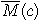. By using (4.2) and (4.3) we obtain: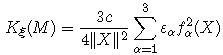If we take now X 2 Γ(D0) we deduce that K»(M) = 0, which proves our assertion.

Theorem 4.2. There are no real lightlike hypersurfaces with positively or negatively Ricci curvature of paraquaternionic space forms.

Proof. Le M be a real lightlike hypersurface of a paraquaternionic space form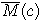. We have: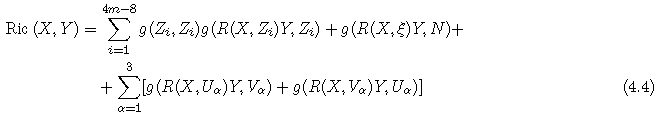where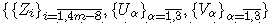is a basis of S(TM)|U. By straightforward computations using (2.3), (2.4), (2.5), (2.6), (4.1) and (4.2) in (4.4) we obtain Ric (X,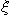) = 0.

#### Example

We consider the paraquaternionic manifold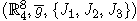where the metric g and the structures J1, J2, J3 are given by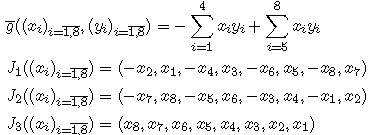We define now a hypersurface M of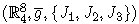by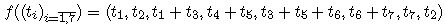Thus the tangent space TM is spanned by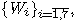where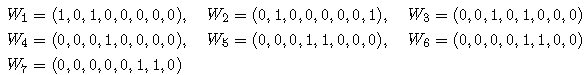If X = (x1, x2, x3, x4, x5, x6, x7, x8) ∈ TM, from conditions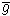(X,Wi) = 0 (i 2 {1, ..., 7}) we obtain

x1 = x3 = x4 = x5 = x6 = x7 = 0, x2 = x8

Hence,

X = (0, x2, 0, 0, 0, 0, 0, x2) = x2W2

Thus TM = Sp(W2) and M is lightlike hypersurface of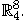#### Acknowledgement

Research was supported by grant D11-22 CEEX 2006-2008.

#### References

Select your language of interest to view the total content in your interested language

### Article Usage

• Total views: 12094
• [From(publication date):
September-2008 - Aug 19, 2019]
• Breakdown by view type
• HTML page views : 8292
• PDF downloads : 3802

## Post your commentCan't read the image? click here to refresh
###### Peer Reviewed Journals

Make the best use of Scientific Research and information from our 700 + peer reviewed, Open Access Journals

International Conferences 2019-20

Meet Inspiring Speakers and Experts at our 3000+ Global Annual Meetings

Top